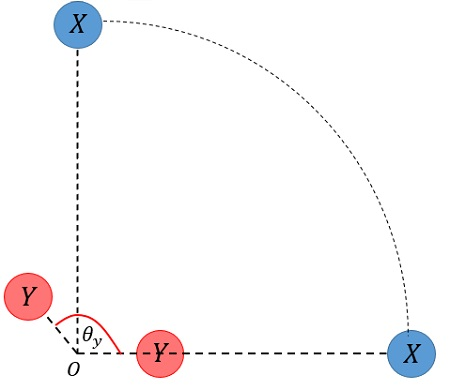# Two stars X and Y travel counterclockwise in circular orbits about a galaxy. The radii of their...

## Question:

Two stars X and Y travel counterclockwise in circular orbits about a galaxy. The radii of their orbits are in the ratio 4:1 . At some time, they are aligned making a straight line with the center of the galaxy. Five years later, star X has rotated through 90 , By what angle has planet Y rotated through during this time?

## Kepler's laws of gravitation

Johannes Kepler (1571-1630) postulated the three laws of planetary motion from extensive observational results. Kepler's laws, as we now know them state:

{eq}\bullet\; 1 {/eq} The planets move in elliptical orbits with the Sun in one focus.

{eq}\bullet\; 2 {/eq} The areolar speed is constant, i.e., the planets in their orbits sweep equal areas in equal times,

{eq}\dfrac{dA}{dt}=constant {/eq}.

{eq}\bullet \; 3 {/eq} The square of the planet's orbital period is proportional to the cube of one half the orbit's major axis.

{eq}T^2 \sim a^3 {/eq}.

The problem considers the orbits to be circular. Stat {eq}X {/eq}'s orbit has a radius {eq}R_X {/eq} The radius of star Y's orbit is,

{eq}R_Y=\dfrac{R_X}{4} {/eq}.

The stars are in a straight line when {eq}\theta=0 {/eq}. We wish to determine the angular position of star Y when star X has rotated through {eq}90^\circ {/eq}. The situation is depicted in the diagram below. The galaxy center is labeled {eq}O {/eq} and the desired angle for star Y is {eq}\theta_Y {/eq}.Using Kepler's third law for the motions of the stars (which we can do for any celestial body rotating about a gravitation center), we can use a law of three relating the orbital periods and radiuses,

{eq}\dfrac{T_X^2}{T_Y^2}=\dfrac{R_X^3}{R_Y^3} {/eq}.

This result can be readily obtained dividing the {eq}T(R) {/eq} relations for each star in Kepler's third law.

Solving for the period of star Y,

{eq}T_Y=\left(\dfrac{R_Y}{R_X}\right)^{3/2}T_X=\dfrac{T_X}{8} {/eq},

since {eq}R_Y/R_X=\dfrac{1}{4} {/eq}.

Consequently, if star X has rotated {eq}90^\circ {/eq} it means it has completed one-quarter of the orbit,

{eq}\Delta t_{90^\circ}=\dfrac{T_X}{4} {/eq}.

{eq}\Delta t_{90^\circ} {/eq} is the total time elapsed since the stars were aligned, substituting it in terms of {eq}T_Y {/eq},

{eq}\Delta t_{90^\circ}=2T_Y {/eq},

the elapsed time corresponds to two full periods of star Y. If one full period is {eq}360^\circ {/eq}, then the angle rotated by star Y is,

{eq}\boxed{\theta_Y=720^\circ} {/eq}.